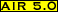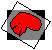# scanair

## Purpose:

Displays the contents of a .air file including the identities of the standard and reslice files and the transformation matrix.

## Usage:

scanair filename [options]

options:
-ldisplays matrix logarithm of the real world transformation matrix
-r
displays the real world transformation matrix in millimeters
-v
displays the voxel coordinate based transformation matrix
where the following definitions apply:
filename
the name of the .air file to be displayed

## Examples:

scanair atlas15.airself
```standard file: atlas15
identifier: 0025690602
file dimensions: 138 by 170 by 143 pixels (x,y,z)
voxel dimensions: 1.000000e+00 by 1.000000e+00 by 1.000000e+00 (x,y,z)

reslice file: atlas15
identifier: 0025690602
file dimensions: 138 by 170 by 143 pixels (x,y,z)
voxel dimensions: 1.000000e+00 by 1.000000e+00 by 1.000000e+00 (x,y,z)

E*cubic standard coordinates=reslice coordinates
E=
[1.000000e+00 0.000000e+00 0.000000e+00 0.000000e+00
0.000000e+00 1.000000e+00 0.000000e+00 0.000000e+00
0.000000e+00 0.000000e+00 1.000000e+00 0.000000e+00
0.000000e+00 0.000000e+00 0.000000e+00 1.000000e+00]

Comment: alignlinear t=(7000 7000) sf=(81 1 3) prec=0.0000 its=25 5 zm=0 pts=1  1 model=6
```
• The .air file atlas15.airself was generated using alignlinear (as indicated in the comment line at the end of the listing). The file atlas15 was used both as the reslice file and as the standard file in order to illustrate the output generated by two files with cubic voxels that are perfectly aligned to one another.
• The identifiers are based upon the same data hashing procedure used by the program identify which can be used to verify that the file atlas15 has not been replaced by another file since this .air file was derived.
scanair atlas10.airself
```standard file: atlas10
identifier: 0024248492
file dimensions: 138 by 170 by 143 pixels (x,y,z)
voxel dimensions: 1.000000e+00 by 1.000000e+00 by 1.750000e+00 (x,y,z)

reslice file: atlas10
identifier: 0024248492
file dimensions: 138 by 170 by 143 pixels (x,y,z)
voxel dimensions: 1.000000e+00 by 1.000000e+00 by 1.750000e+00 (x,y,z)

E*cubic standard coordinates=reslice coordinates
E=
[1.000000e+00 0.000000e+00 0.000000e+00 0.000000e+00
0.000000e+00 1.000000e+00 0.000000e+00 0.000000e+00
0.000000e+00 0.000000e+00 5.714286e-01 0.000000e+00
0.000000e+00 0.000000e+00 0.000000e+00 1.000000e+00]

Comment: alignlinear t=(7000 7000) sf=(81 1 3) prec=0.0000 its=25 5 zm=0 pts=1  1 model=6
```
• The .air file atlas10.airself was generated using alignlinear (as indicated in the comment line at the end of the listing). The file atlas10 was used both as the reslice file and as the standard file in order to illustrate the output generated by two files with non-cubic voxels that are perfectly aligned to one another.
• The term 5.714286e-01 in this example is not an error. This term is not 1.000000e+00 because the parameters in an .air file are defined such that the reslice file will match a version of the standard file that has been interpolated to cubic voxels. The value 5.714286e-01=1/1.75 makes the adjustment necessary for matching the interpolated file.
scanair atlas10.airself -k
```standard file: atlas10
identifier: 0024248492
file dimensions: 138 by 170 by 143 pixels (x,y,z)
voxel dimensions: 1.000000e+00 by 1.000000e+00 by 1.750000e+00 (x,y,z)

reslice file: atlas10
identifier: 0024248492
file dimensions: 138 by 170 by 143 pixels (x,y,z)
voxel dimensions: 1.000000e+00 by 1.000000e+00 by 1.750000e+00 (x,y,z)

R*standard spatial location=reslice spatial location
R=
[1.000000e+00 0.000000e+00 0.000000e+00 0.000000e+00
0.000000e+00 1.000000e+00 0.000000e+00 0.000000e+00
0.000000e+00 0.000000e+00 1.000000e+00 0.000000e+00
0.000000e+00 0.000000e+00 0.000000e+00 1.000000e+00]

Comment: alignlinear t=(7000 7000) sf=(81 1 3) prec=0.0000 its=25 5 zm=0 pts=1  1 model=6

```
• This is identical to the previous example except that the -r flag is used. As a result, the real world matrix provided here correctly shows that the images are perfectly aligned. If you are interested in determining real world characteristics of a .air file (e.g., whether it describes a rigid-body deformation, the -r option (or -l option) should be used.

• The 4x4 matrix R is a homogenous coordinate transformation matrix.
• Note that coordinates are in the internal coordinate system used by the AIR package and may not correspond in a straightforward way to coordinates generated by your image display package.
• The contents of the comment line will vary according to the program that generated the .air file. The first item in the comment line will generally be the name of the program that generated the .air file.
•The -l flag will give a matrix that is the matrix logarithm of the real world transformation matrix. This matrix logarithm should not be used for coordinate transformations, but describes an instantaneous movement produced by the original matrix. Rigid-body transformations will generate anti-symmetric matrices with zero's along the diagonal, making this a quick way to diagnose whether a given matrix describes a rigid-body transformation.

## Error messages: (alphabetical by case)Modified: December 17, 2001